# WEEKLY MOCK-NEW BATCH

Cell

River

Dice

Municipality

Preamble

Ratio

Transport

Fiscal policy

Md Ghori & Iltutmish

1. This cell is the longest in the human body
2. This structure of the plant cell is non-living
3. Animal cells are interconnected by
4. The Cell theory is not applicable to
5. This tissue includes the blood tissue
6. Hirakud Hydel Power station is located on which River?
7. Consider the following river of India:
1. Krishna River
2. Cauvery River
3. Godavari River
Which among the above originate in Western Ghats?
8. Gandak river is associated with one of the following river systems?
9. Kharkai is the tributary of which of the following river?
10. Which river is known as "Sorrow of Bengal"?
11.
 Which digit will appear on the face opposite to the face with number 4?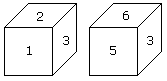12.
 Which symbol will be on the face opposite to the face with symbol * ?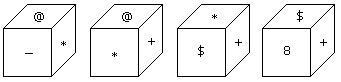13.
 Two positions of dice are shown below. How many points will appear on the opposite to the face containing 5 points?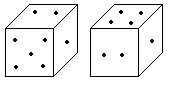14.
 How many points will be on the face opposite to in face which contains 2 points?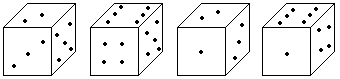15.
 Which number is on the face opposite to 6?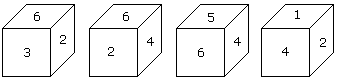16. Two positions of a dice are shown below. When number '1' is on the top. What number will be at the bottom?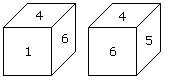17.
 Here two positions of dice are shown. If there are two dots in the bottom, then how many dots will be on the top?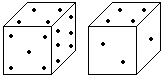18.   Which of the following are functional items in the Twelfth Schedule of the Constitution?1) Roads and bridges.
2) Urban forestry.
3) Urban poverty alleviation.
4) Non-conventional energy sources.
5) Regulation of land use.
19. Where was the first Municipal Corporation set up in India?
20.  Central Council of Local Government was setup in -
21. Which of the following word has not been written in the preamble of the Indian Constitution?
22. Which of the following statements is not true?
23. Which statement is not correct in the case of "Sovereign India"?
24. K.M. Munshi was related to......
25. What is the true meaning of "Secular"?
26. What is the meaning of "social equality" in the Indian Constitution?
27. Who among the following said that the preamble of the Indian Constitution is "The Keynote of the Constitution"?
28. "The language of Preamble" of the Indian constitution is taken from the constitution of......
29. Rs. 8400 is divided among A, B, C and D in such a way that the shares of A and B, B and C, and C and D are in the ratios of 2:3, 4:5 and 6:7 respectively. The share of A is
30. The ratio of the present age of father to that of son is 7:2. After 10 years their ages will be in the ratio of 9:4. The present ages of the father is
31.  Ajay and Raj together have Rs. 1050. On taking Rs. 150 from Ajay, Ajay will have same amount as what Raj had earlier. Find the ratio of amounts with Ajay and Raj initially.
32. The ratio of market prices of wheat and paddy is 2:3 and the ratio of quantities consumed in a family is 5:4. Find the ratio of expenditure of wheat and paddy.
33. The ratio of numbers of girls and boys participating in sports of a school is 4:5. If the number of girls is 212, determine the number of boys participating in the sports.
34. The three numbers are in the ratio 1/2 : 2/3 : 3/4. The difference between greatest and smallest numbers is 36. Find the numbers.
35. If A:B = 2:3, B:C = 4:5 and C:D = 6:7, then A:B:C:D is
36. If x:y = 3:4, then (7x+3y):(7x-3y) is equal to
37. The product of two positive numbers is 4752 and their ratio is 11:12. The smaller of these numbers is
38. a:b = 3:7 and b:c = 9:5. What is a:b:c?
39. Who is responsible for the construction and maintenance of the National Highways?
40. When was the Border Roads Development Organisation (BRO) set up?
41. When was Pawan Hans Ltd. introduced?
42. When was International Airport Authority of India (IAAI) set up?
43. Which is the biggest port in the country?
44.  Which is the highest airport in India?
45.  Which was the first railway terminus in India?
46. Which was the first superfast train in India?
47. Which was the first double decker train in India?